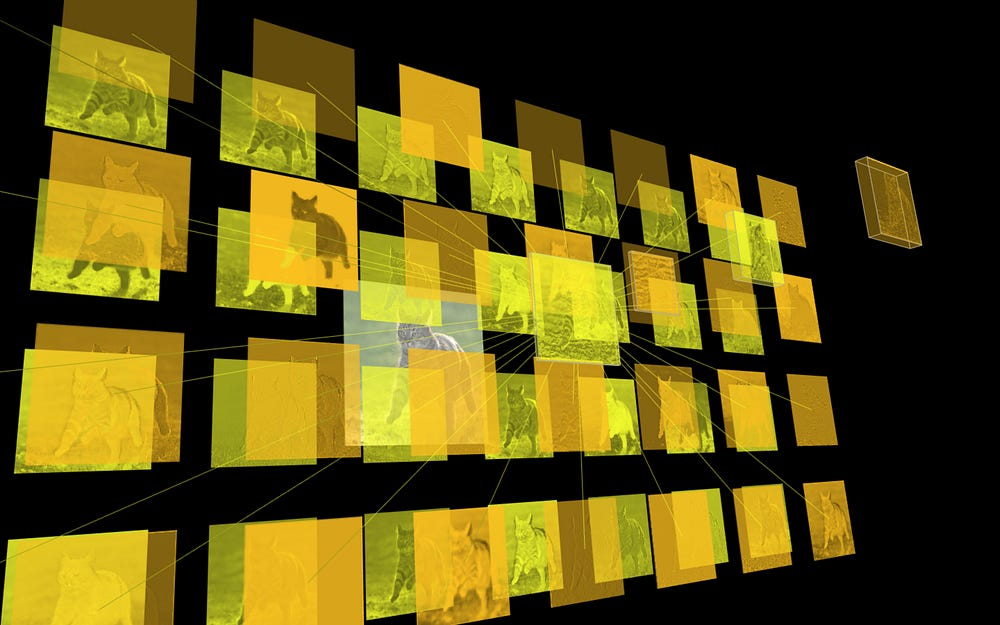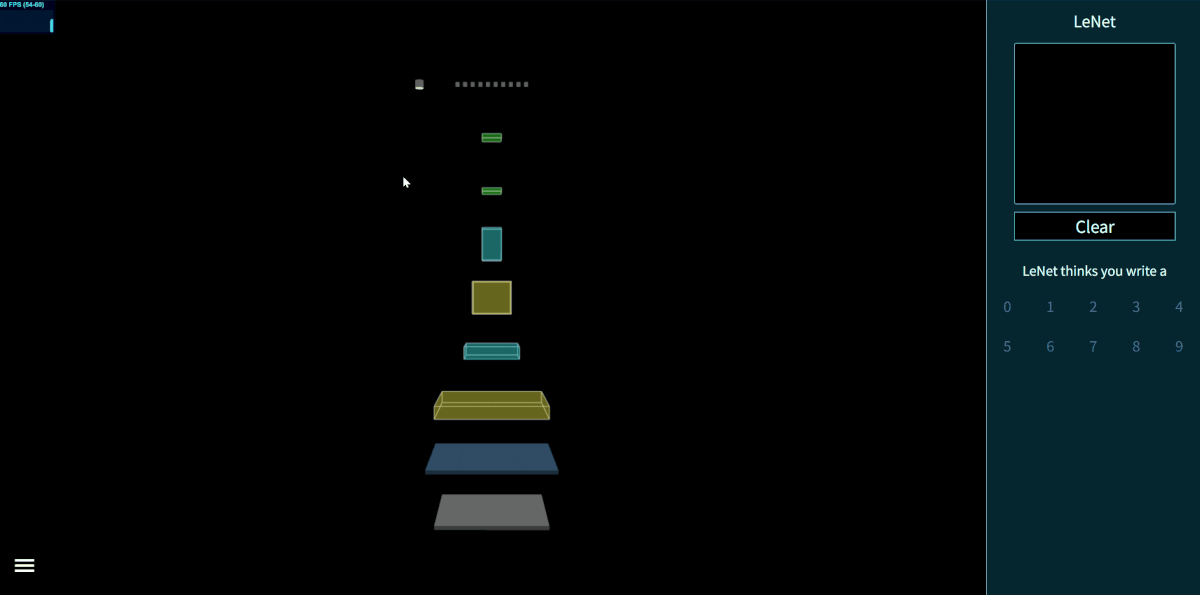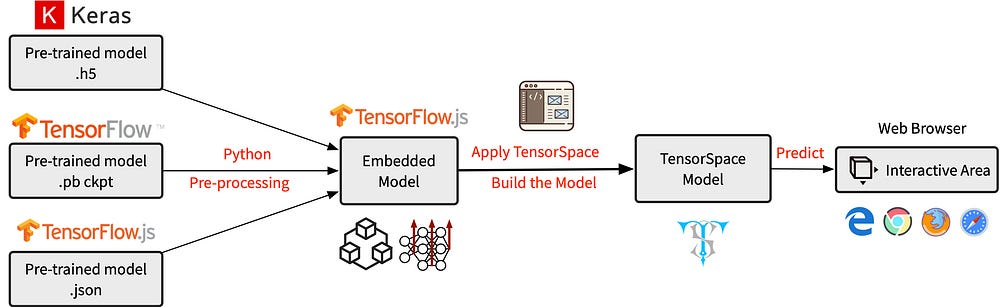TensorSpace.js — A Way to 3D Visualize Neural Networks in Browsers

Source: Deep Learning on MediumFeature Abstractions of a Tiger Cat Image

The neural networks were something high-level, unreachable and mysterious before I took my first deep learning class. To me they are just magics: the neural network applications can complete tasks on the object detection, the image classification and even the data prediction in our daily lives.

“What does the model compute?”, “Why should we use this specific network for this task?”, “How could others come up the structure like this?”

Maybe you have the same questions as I have. My friends and I find sometimes, it is really hard to understand and explain the neural networks. Then some ideas come up: “why not to visualize a neural network?”, “how about a 3D model?”, “it can be interactive!”. Since there is no such a wheel existing, why not create by ourselves? After 6 months, I am proud to introduce our effort — TensorSpace.js.

What is TensorSpace.js?Fig. 1 — Interactive LeNet model created by TensorSpace.js

TensorSpace.js is a neural network 3D visualization framework built by TensorFlow.js, Three.js and Tween.js.

Since we want to easily present the models anywhere, that is, in most web browsers, we choose JavaScript to implement the framework. From the Fig. 1 above, we can easily check the model structure: each “cube” represents a “layer” object in the neural network. Next, we can actually interact with the model by clicking, dragging and scrolling. Different angles may provide different view points of the model. Some objects are expandable, which allows us to observe more details. Further more, we design a hybrid architecture for TensorSpace.js to support different libraries, such as TensorFlow, Keras, TensorFlow.js (more in the future).

TensorSpace.js can not only show the basic model structure, but also present the processes of internal feature abstractions, intermediate data manipulations and final inference generations.

In summary, TensorSpace.js is:

• Interactive — Use Keras-like API to build interactive model in browsers.
• Intuitive — Visualize the information from intermediate inferences.
• Integrative — Support pre-trained models from TensorFlow, Keras, TensorFlow.js.

How does TensorSpace.js work?

The following part introduces how to build a TensorSpace model. I use an LeNet handwritten recognition model as an example. You can find the all example files from the repo: TensorSpace — HelloWorld.Fig. 2 — Workflow to present a TensorSpace model

The general workflow is to create or preprocess a pre-trained model with multiple intermediate outputs. Then based on the neural network structure, we can build a TensorSpace model. Last, we load and initialize the model which can accept input data for inferences.

After correctly installing TensorSpace.js and properly preprocessing the pre-trained models, we can easily create an LeNet handwritten recognition TensorSpace model. For convenience, we use the preprocessed TensorSpace compatible model and an extracted file which is a handwritten “5” as the model input.

let container = document.getElementById( "container" );
// Create sequential model
let model = new TSP.models.Sequential( container );
// Add LeNet Layers
model.add( new TSP.layers.GreyscaleInput({ shape: [28, 28, 1] }) );
model.add( new TSP.layers.Conv2d({ kernelSize: 5, filters: 6, strides: 1 }) );
model.add( new TSP.layers.Pooling2d({ poolSize: [2, 2], strides: [2, 2] }) );
model.add( new TSP.layers.Conv2d({ kernelSize: 5, filters: 16, strides: 1 }) );
model.add( new TSP.layers.Pooling2d({ poolSize: [2, 2], strides: [2, 2] }) );
model.add( new TSP.layers.Dense({ units: 120 }) );
model.add( new TSP.layers.Dense({ units: 84 }) );
units: 10,
outputs: ["0", "1", "2", "3", "4", "5", "6", "7", "8", "9"]
}) );
// Load preprocessed model
type: "tfjs",
url: './lenetModel/mnist.json'
});
// Initialize the model in the browser
model.init(function() {
// Predict input "5"
model.predict( image_5 );
});

That’s it!

You can try in the CodePen:

It is easy to build other preprocessed models in the same way. If you are interested, please check our playground for more interesting demos, such as Yolov2-tiny, ACGAN, and ResNet-50.

When to use?

If you want to present your model to others, if you want to explain some detailed features of the model, if you want to build a demo from scratch, I think TensorSpace can be a good tool to describe the model intuitively and clearly. It is fun to interact with and explore the model you just built.

Conclusion

My team and I hope TensorSpace can, at least, help to move a little step forward on how to visualize the neural networks, which can attract more people to be interested in this field.

For further information about TensorSpace.js, please check: